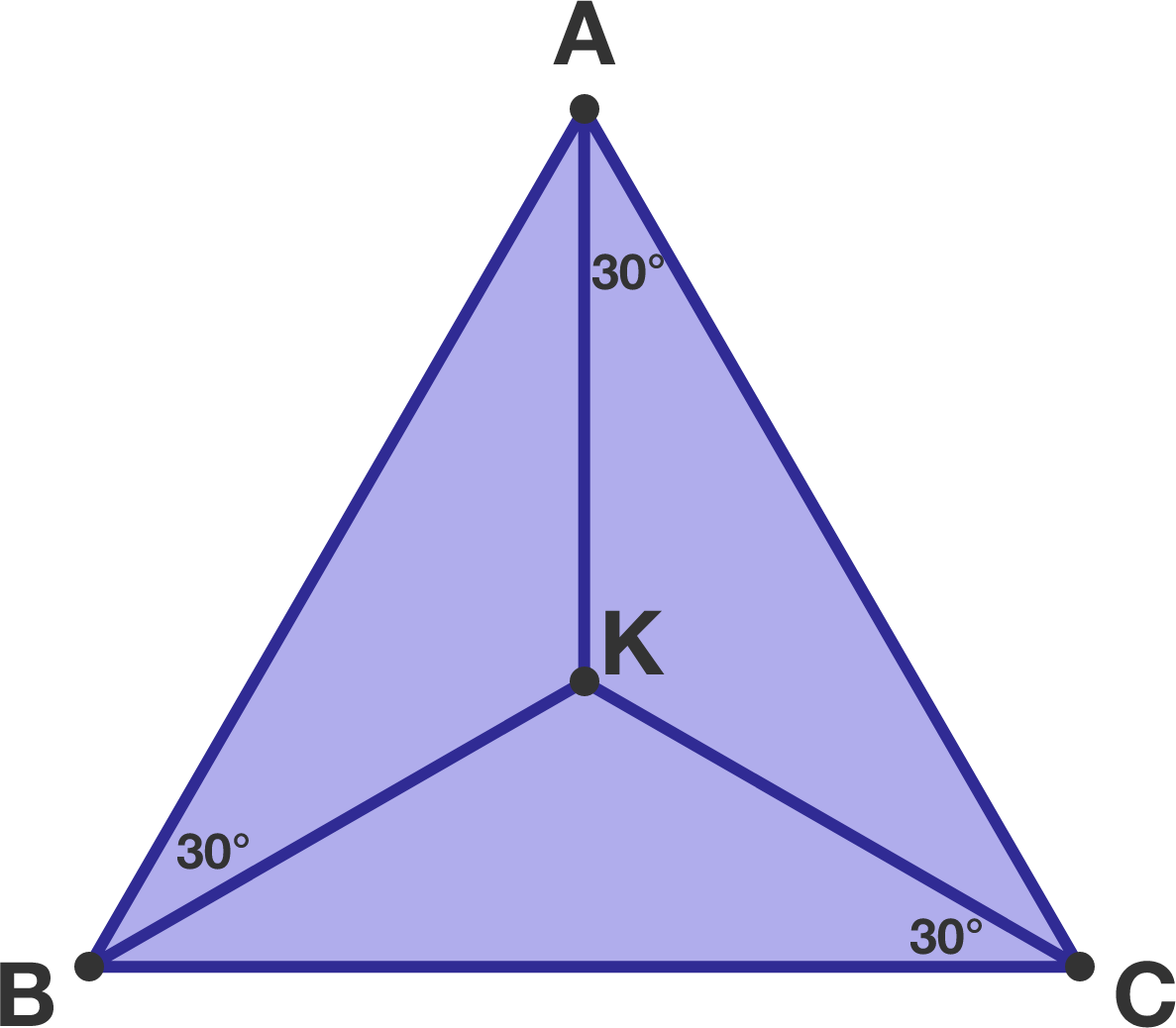# Equilateral triangle?

Geometry Level 1In triangle $ABC,$ there exists a point $K$ inside of it such that

$\angle{ABK}= \angle{BCK}= \angle{CAK}=30^\circ.$

Is it necessarily true that triangle $ABC$ is equilateral?

×

Problem Loading...

Note Loading...

Set Loading...